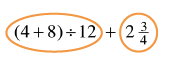### Home > CC1 > Chapter 8 > Lesson 8.1.4 > Problem8-52

8-52.

Simplify each of the following expressions.  Homework Help ✎

For parts (a), (b), and (c), remember to circle the terms. A term is a single number or a product of numbers.
Simplify each term until it is a single number. Then combine terms. Don't forget the Order of Operations. It is very important.

1. $(4+8)\div12+2\frac{3}{4}$

Try circling each term to start, then simplify from left to right.$3\frac{3}{4}$

1. $3·(8−5)+6+2·7$

Be sure to simplify the expression inside of the parentheses before multiplying from left to right.

Make sure you carry out each multiplication operation before using addition! The terms are circled above.

1. $49\div7·5+4·(3.13+2.12)$

Have you circled the terms?

Make sure you followed the Order of Operations closely! It is important to multiply and divide from left to right, or you may simplify the expression incorrectly.

$56$